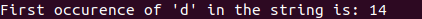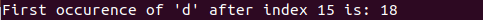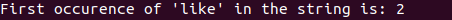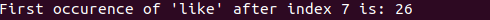# Java String indexOf() Function: Complete Guide

A common scenario can be when a system admin wants to find the index of the ‘@’ character of the email Id of a client and then wants to get the remaining substring. In that situation, the IndexOf() method can be used.

## Java String indexOf()

Java string indexOf() is a built-in method that returns the index of a given character value or substring. If it is not found, then it returns -1. The index counter starts from zero. The indexOf() method is used to get the integer value of a particular index of String type object, based on criteria specified in the parameters of the indexOf() method.

## Types of indexOf method

### String indexOf( char ch):

The indexOf(char ch) method returns the index of the first occurrence of the given character; if it is not present there, it returns -1.

#### Syntax

```stringName.indexOf(character)
```

Here stringName is the string from which you want to find the index of the character, and character means that character whose index you want to find.

See the following program.

```class IndexOfStr {
public static void main(String args[]) {
String str = "Welcome to Appdividend";
System.out.print("First occurence of 'd' in the string is: ");
// we are finding the index of 'd'
// it will return the index of first
// occurence of 'd'
System.out.println(str.indexOf('d'));

}
}```

See the output.### String indexOf(char ch, int x)

The indexOf() method returns an index of the given character present in a string, but it searches its position at index x. If it cannot find the index of the character, it returns -1.

#### Syntax

```int indexOf(int ch, int start)
```

Here ch is the character whose position we want to find, and start is the index starting from where we want to find the index of ch.

See the following code.

```class IndexOfStr {
public static void main(String args[]) {
String str = "Welcome to Appdividend";
System.out.print("First occurence of 'd' after index 15 is: ");
// we are finding the index of 'd'
// it will return the index of d
// after index position 15
System.out.println(str.indexOf('d', 15));

}
}```

See the output.### String indexOf(String str)

The indexOf(String str) method returns an index of the first occurrence of the substring from a given string.

#### Syntax

```int indexOf(String str)
```

Here str is the substring whose index we want to find from the given string.

```class IndexOfStr {
public static void main(String args[]) {
String str = "I like Apple. What do you like?";
System.out.print("First occurence of 'like' in the string is: ");
// Initialising search string
String substr = new String("like");
// we are finding the index of 'like'
// it will return the index of first
// occurence of 'like'
System.out.println(str.indexOf(substr));

}
}```

See the output.### String indexOf(String str, int x)

The indexOf() method returns the index of the given substring but starts from the given index x. After index position x, it will return the first occurrence of the given substring.

#### Syntax

```int indexOf(String str, int x)
```

Here str is the character whose position we want to find, and x is the index starting from where we want to find the index of str.

See the program.

```class IndexOfStr {
public static void main(String args[]) {
String str = "I like Apple. What do you like?";
System.out.print("First occurence of 'like' after index 7 is: ");
// Initialising search string
String substr = new String("like");
// we are finding the index of 'like'
// it will return the index of first
// occurence of 'like'
System.out.println(str.indexOf(substr, 7));

}
}```

See the output.That’s it for this tutorial.

## Recommended Posts

Java String compareTo()

How to find string length In Java

Java String toUpperCase()

Java Trim String Example

Java String valueOf()

This site uses Akismet to reduce spam. Learn how your comment data is processed.